CATEGORIES:

# Mechanical Energy

Let a body be acted upon by a number of forces whose vector sum is equal to zero. The body moves uniformly and rectilinearly. All the forces may than be resolved into four components.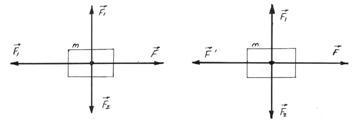Figure 5.1(5.4)

Forcesand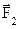in accordance with the adopted definition, perform no work. Force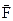performs work equal to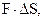where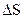is the traversed distance. The work of force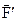is equal to, where the minus sing indicates that work is performed against the force.

Let us now consider the motion of a body with acceleration (curvilinear and non uniform motion). Now,is not equal to, andis not equal to. The work of forceis again negative, i.e., the work is performed against the forceand equalThe inequality between the forcesandshows that the motion is curvilinear. Their differencecorresponds to the normal component of the acceleration. Let us consider an extreme case – uniform motion in a circle. The resultant force for such motion is directed, as we know, along the radius of the circle, i.e., perpendicular to the direction of motion. Therefore, the centripetal force performs no work.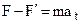is the tangential component of the resultant force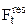, and(5.5)

The work expended in accelerating a body is equal to the product of the mass of the body, the traversed distance, and the tangential acceleration. The work performed by the force of resistance and the work expended in accelerating the body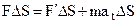(5.6)

For, we obtain; (5.7)

where v is the average velocity, which is equal(5.8)

Since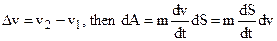or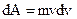. In result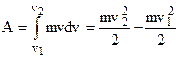. (5.9)

Date: 2015-01-12; view: 999

 <== previous page | next page ==> Motion in circle is accelerated motion | Kinetic Energy and the Work-Energy Theorem
doclecture.net - lectures - 2014-2023 year. Copyright infringement or personal data (0.011 sec.)